### Answers − Quadratic Graphs using x and y axis − 2

#### 1.   Draw the graph y = 2x2 + 10x + 8

 (a) The graph crosses the y axis at the number term c which is 8. When x = 0 then y = 8. The coordinate is (0, 8)
 (b) The graph crosses the x axis where 2x2 + 10x + 8 = 0. Refer to question 1 of Solve Quadratics by Factorising - 2. So y = 0 when x = −4 or −1.The coordinates are (−4, 0) and (−1, 0)
 (c) Draw the graph using the above coordinates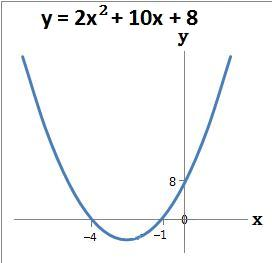#### 2.   Draw the graph y = 3x2 + 21x + 18

 (a) The graph crosses the y axis at the number term c which is 18. When x = 0 then y = 18. The coordinate is (0, 18)
 (b) The graph crosses the x axis where 3x2 + 21x + 18 = 0. Refer to question 2 of Solve Quadratics by Factorising - 2. So y = 0 when x = −6 or −1.The coordinates are (−6, 0) and (−1, 0)
 (c) Draw the graph using the above coordinates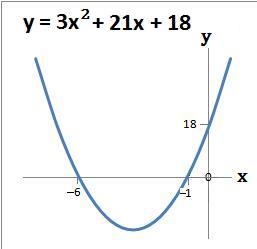#### 3.   Draw the graph y = 4x2 + 20x − 24

 (a) The graph crosses the y axis at the number term c which is −24. When x = 0 then y = −24. The coordinate is (0, −24)
 (b) The graph crosses the x axis where 4x2 + 20x − 24 = 0. Refer to question 3 of Solve Quadratics by Factorising - 2. So y = 0 when x = −6 or 1.The coordinates are (−6, 0) and (1, 0)
 (c) Draw the graph using the above coordinates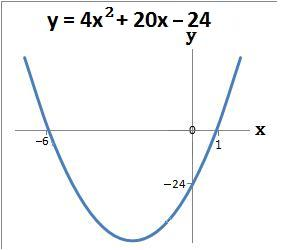#### 4.   Draw the graph y = 2x2 + 9x − 56

 (a) The graph crosses the y axis at the number term c which is −56. When x = 0 then y = −56. The coordinate is (0, −56)
 (b) The graph crosses the x axis where 2x2 + 9x − 56 = 0. Refer to question 4 of Solve Quadratics by Factorising - 2. So y = 0 when x = −8 or 7/2.The coordinates are (−8, 0) and (7/2, 0)
 (c) Draw the graph using the above coordinates#### 5.   Draw the graph y = 5x2 − 20x − 60

 (a) The graph crosses the y axis at the number term c which is −60. When x = 0 then y = −60. The coordinate is (0, −60)
 (b) The graph crosses the x axis where 5x2 − 20x − 60 = 0. Refer to question 5 of Solve Quadratics by Factorising - 2. So y = 0 when x = −2 or 6.The coordinates are (−2, 0) and (6, 0)
 (c) Draw the graph using the above coordinates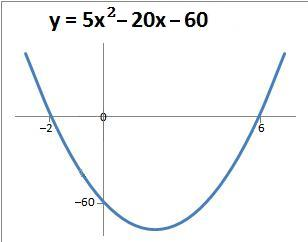#### 6.   Draw the graph y = 3x2 − 21x + 36

 (a) The graph crosses the y axis at the number term c which is 36. When x = 0 then y = 36. The coordinate is (0, 36)
 (b) The graph crosses the x axis where 3x2 − 21x + 36 = 0. Refer to question 6 of Solve Quadratics by Factorising - 2. So y = 0 when x = 3 or 4.The coordinates are (3, 0) and (4, 0)
 (c) Draw the graph using the above coordinates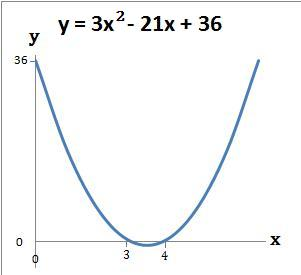#### 7.   Draw the graph y = 3x2 − 40x + 48

 (a) The graph crosses the y axis at the number term c which is 48. When x = 0 then y = 48. The coordinate is (0, 48)
 (b) The graph crosses the x axis where 3x2 − 40x + 48 = 0. Refer to question 7 of Solve Quadratics by Factorising - 2. So y = 0 when x = 4/3 or 12.The coordinates are (4/3, 0) and (12, 0)
 (c) Draw the graph using the above coordinates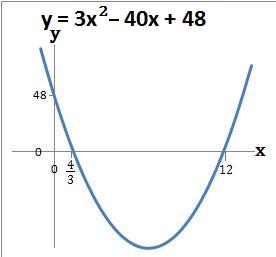back to: